# 978-0073382395 Chapter 2 Questions and Problems

Document Type
Homework Help
Book Title
Fundamentals of Corporate Finance Standard Edition 9th Edition
Authors
Stephen Ross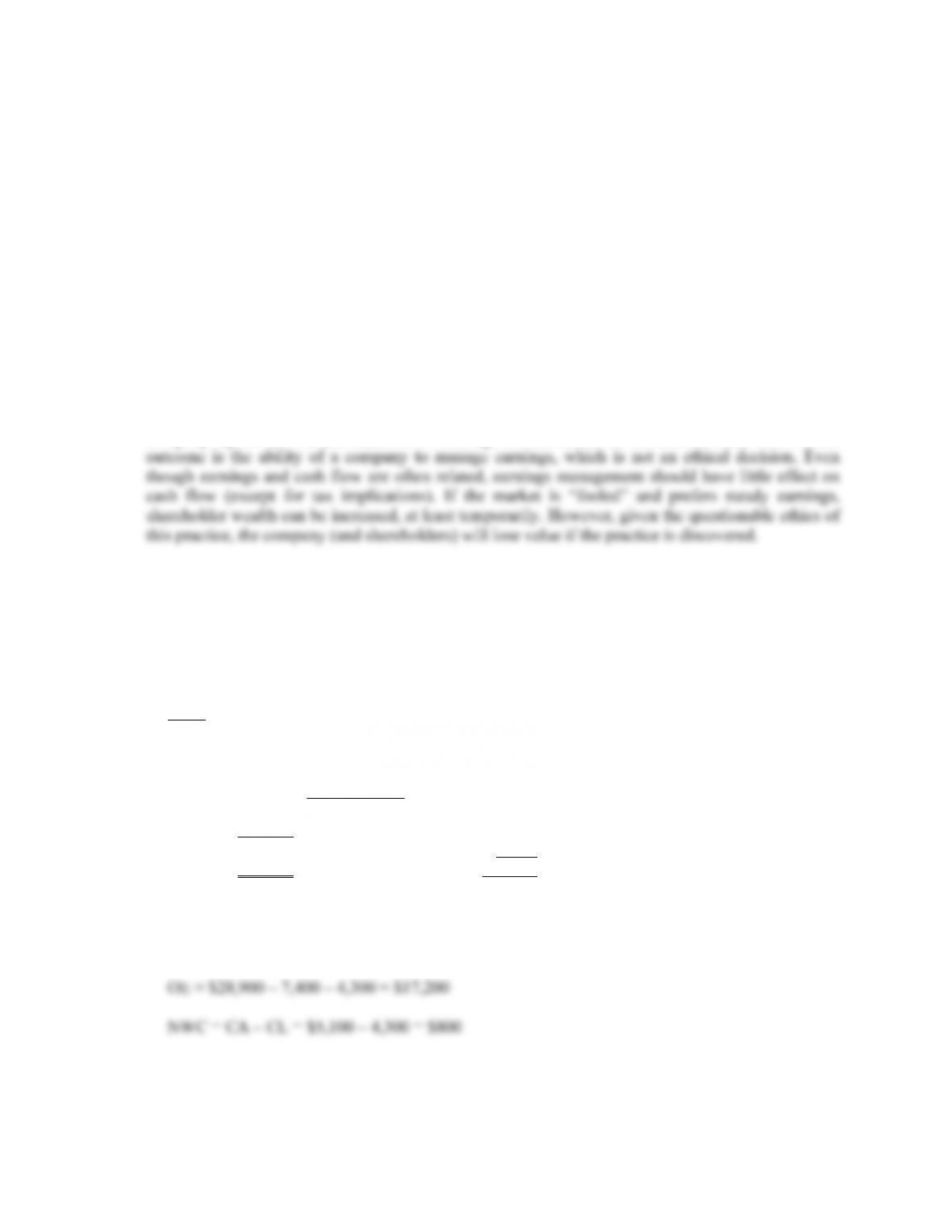CHAPTER 2 B-5
9. If a company raises more money from selling stock than it pays in dividends in a particular period,
its cash flow to stockholders will be negative. If a company borrows more than it pays in interest, its
cash flow to creditors will be negative.
10. The adjustments discussed were purely accounting changes; they had no cash flow or market value
consequences unless the new accounting information caused stockholders to revalue the derivatives.
11. Enterprise value is the theoretical takeover price. In the event of a takeover, an acquirer would have
to take on the company's debt, but would pocket its cash. Enterprise value differs significantly from
simple market capitalization in several ways, and it may be a more accurate representation of a firm's
value. In a takeover, the value of a firm's debt would need to be paid by the buyer when taking over
a company. This enterprise value provides a much more accurate takeover valuation because it
includes debt in its value calculation.
12. In general, it appears that investors prefer companies that have a steady earnings stream. If true, this
encourages companies to manage earnings. Under GAAP, there are numerous choices for the way a
company reports its financial statements. Although not the reason for the choices under GAAP, one
Solutions to Questions and Problems
NOTE: All end of chapter problems were solved using a spreadsheet. Many problems require multiple
steps. Due to space and readability constraints, when these intermediate steps are included in this
solutions manual, rounding may appear to have occurred. However, the final answer for each problem is
found without rounding during any step in the problem.
Basic
1. To find owner’s equity, we must construct a balance sheet as follows:
Balance Sheet
CA \$5,100 CL \$4,300
NFA 23,800 LTD 7,400
OE ??
TA \$28,900 TL & OE \$28,900
We know that total liabilities and owner’s equity (TL & OE) must equal total assets of \$28,900.
We also know that TL & OE is equal to current liabilities plus long-term debt plus owner’s
equity, so owner’s equity is: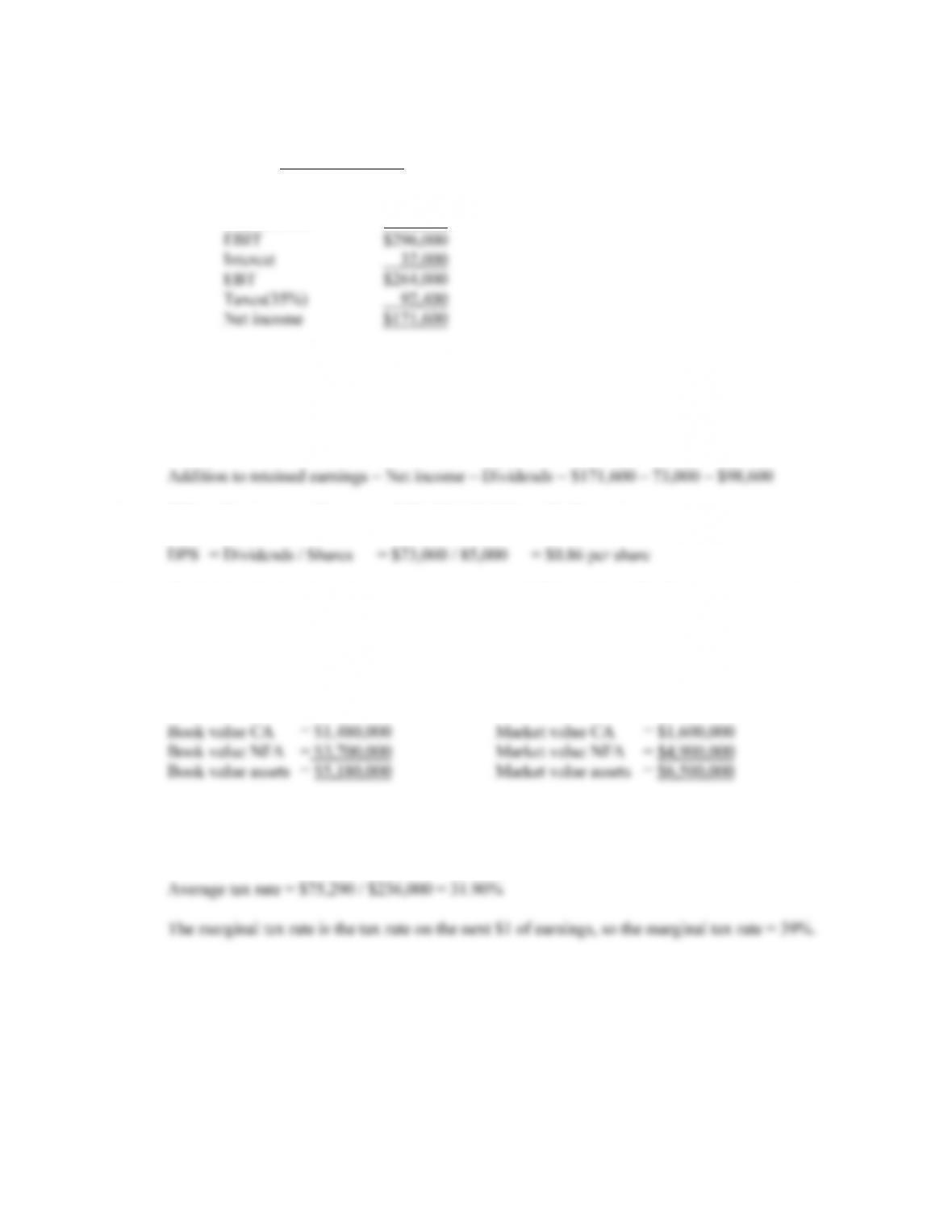B-6 SOLUTIONS
2. The income statement for the company is:
Income Statement
Sales \$586,000
Costs 247,000
Depreciation 43,000
3. One equation for net income is:
Net income = Dividends + Addition to retained earnings
Rearranging, we get:
4. EPS = Net income / Shares = \$171,600 / 85,000 = \$2.02 per share
5. To find the book value of current assets, we use: NWC = CA – CL. Rearranging to solve for
current assets, we get:
CA = NWC + CL = \$380,000 + 1,400,000 = \$1,480,000
The market value of current assets and fixed assets is given, so:
6. Taxes = 0.15(\$50K) + 0.25(\$25K) + 0.34(\$25K) + 0.39(\$236K – 100K) = \$75,290
7. The average tax rate is the total tax paid divided by net income, so: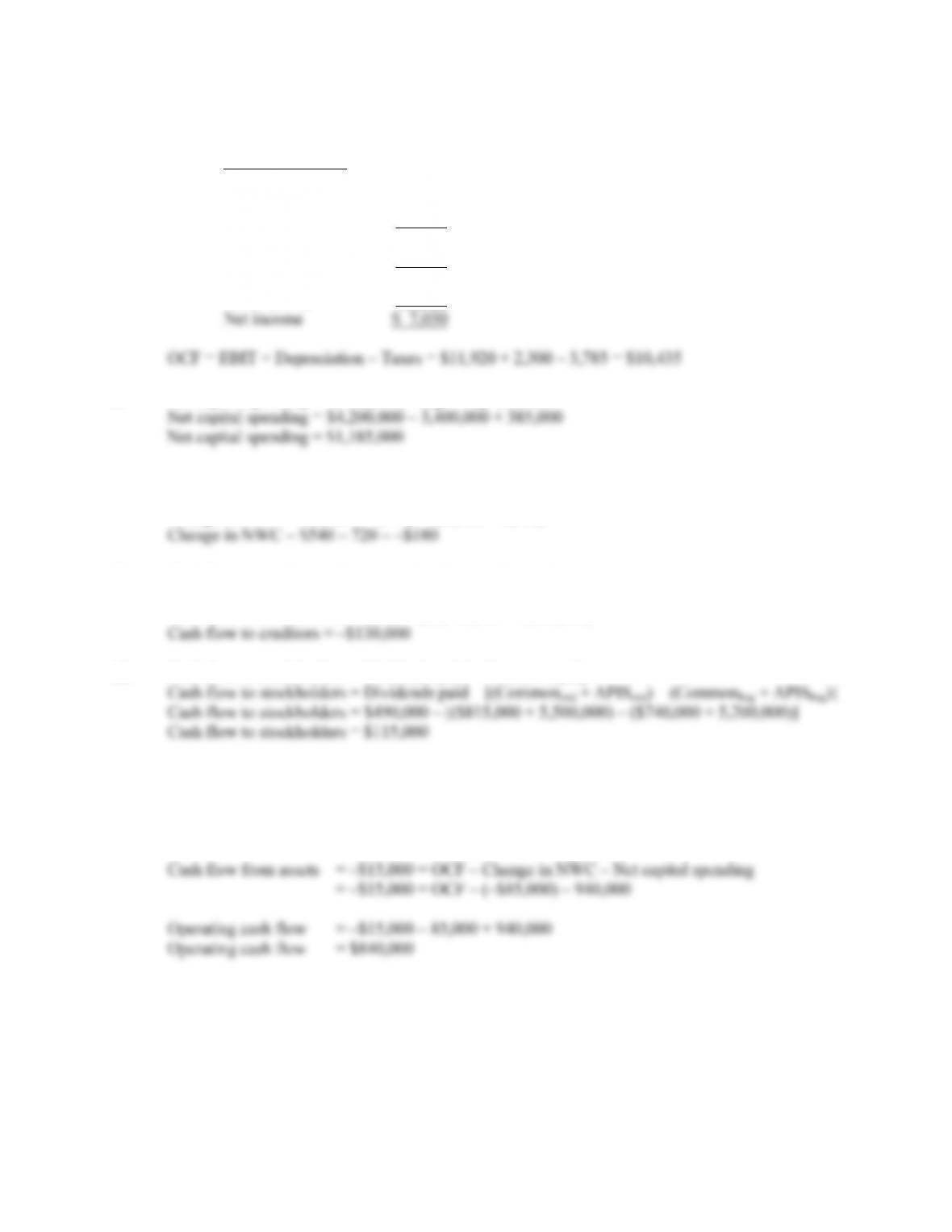CHAPTER 2 B-7
8. To calculate OCF, we first need the income statement:
Income Statement
Sales \$27,500
Costs 13,280
Depreciation 2,300
EBIT \$11,920
Interest 1,105
Taxable income \$10,815
Taxes (35%) 3,785
9. Net capital spending = NFAend – NFAbeg + Depreciation
10. Change in NWC = NWCend – NWCbeg
Change in NWC = (CAend – CLend) – (CAbeg – CLbeg)
Change in NWC = (\$2,250 – 1,710) – (\$2,100 – 1,380)
11. Cash flow to creditors = Interest paid – Net new borrowing
Cash flow to creditors = Interest paid – (LTDend – LTDbeg)
Cash flow to creditors = \$170,000 – (\$2,900,000 – 2,600,000)
12. Cash flow to stockholders = Dividends paid – Net new equity
Note, APIS is the additional paid-in surplus.
13. Cash flow from assets = Cash flow to creditors + Cash flow to stockholders
= –\$130,000 + 115,000 = –\$15,000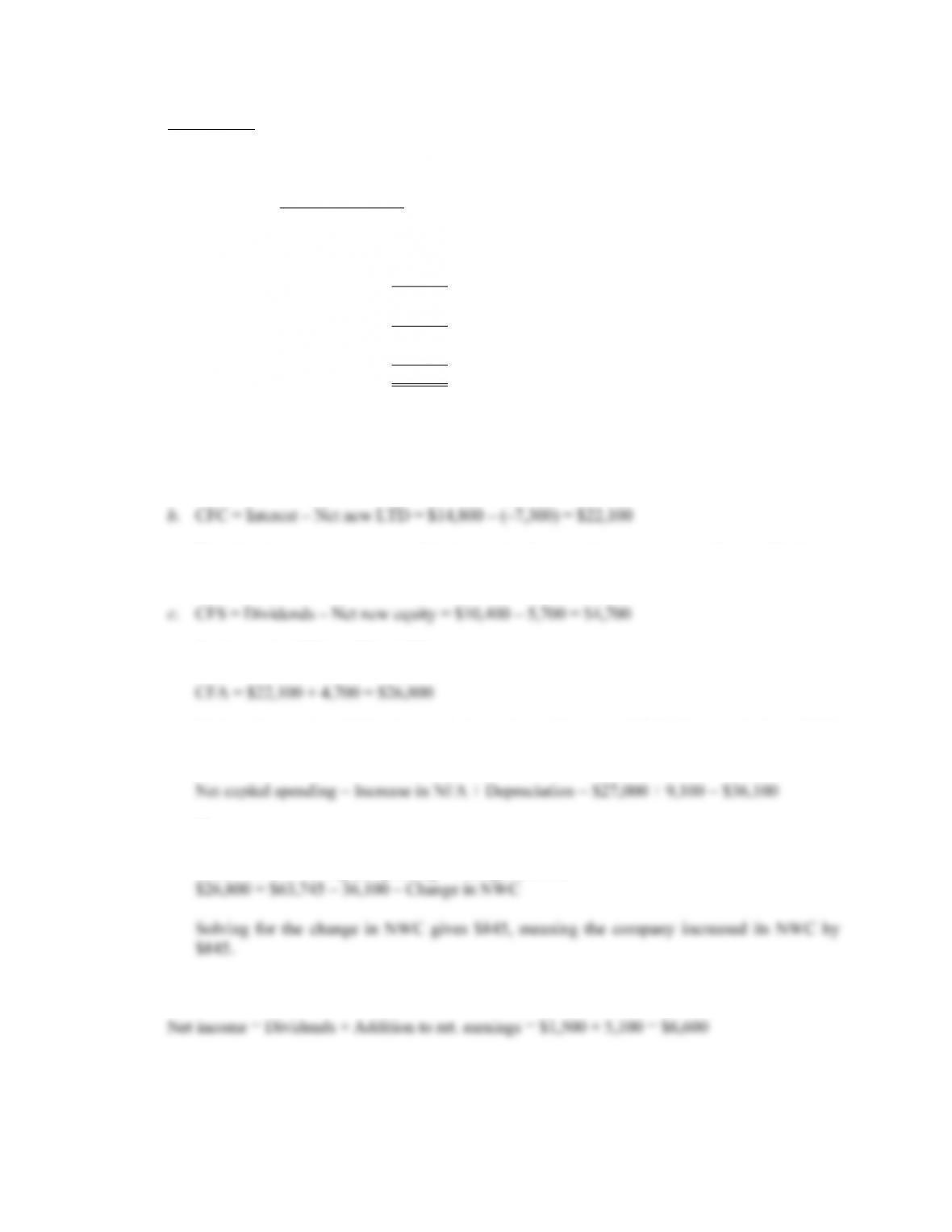B-8 SOLUTIONS
Intermediate
14. To find the OCF, we first calculate net income.
Income Statement
Sales \$196,000
Costs 104,000
Other expenses 6,800
Depreciation 9,100
EBIT \$76,100
Interest 14,800
Taxable income \$61,300
Taxes 21,455
Net income \$39,845
Dividends \$10,400
a. OCF = EBIT + Depreciation – Taxes = \$76,100 + 9,100 – 21,455 = \$63,745
Note that the net new long-term debt is negative because the company repaid part of its long-
term debt.
d. We know that CFA = CFC + CFS, so:
CFA is also equal to OCF – Net capital spending – Change in NWC. We already know OCF.
Net capital spending is equal to:
Now we can use:
CFA = OCF – Net capital spending – Change in NWC
15. The solution to this question works the income statement backwards. Starting at the bottom: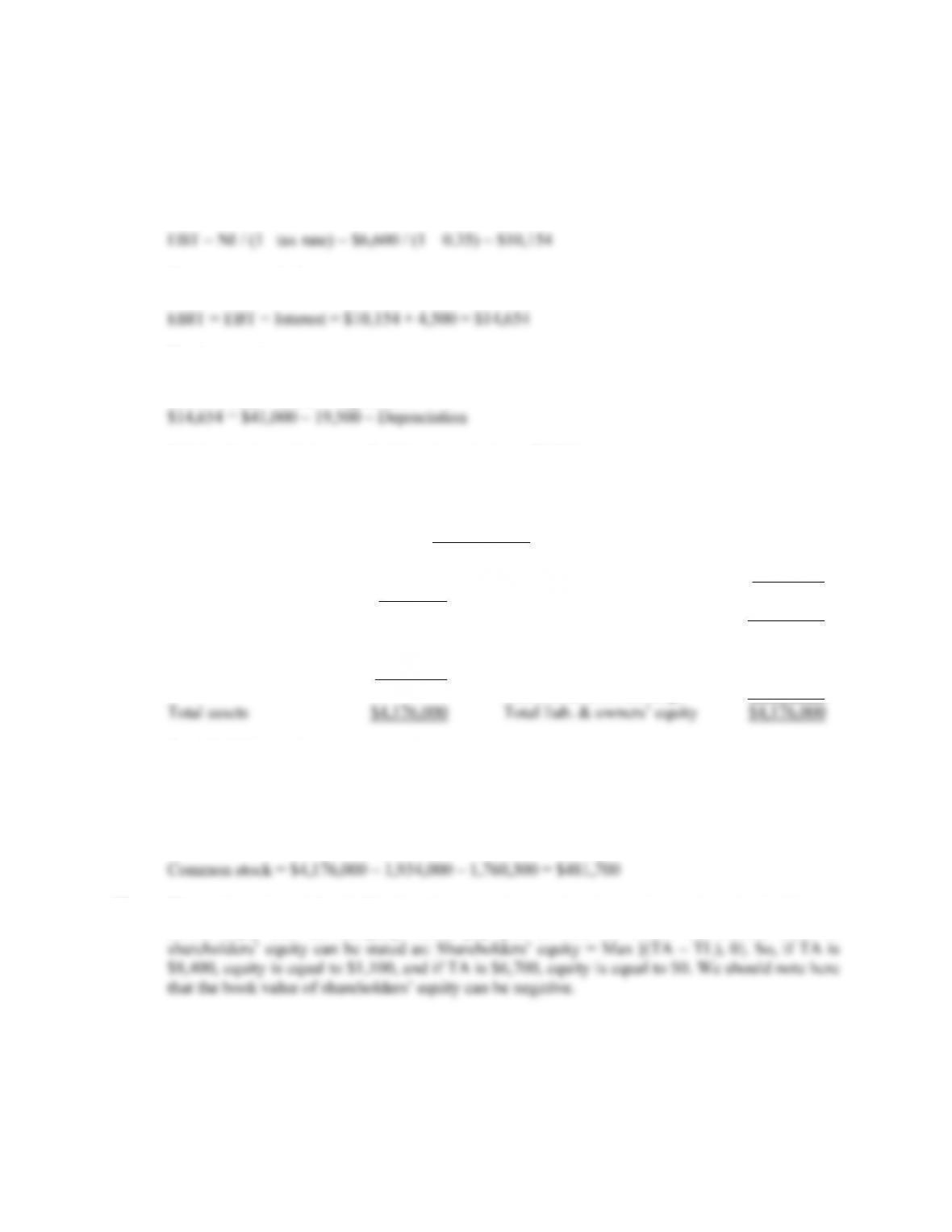CHAPTER 2 B-9
Now, looking at the income statement:
EBT – EBT × Tax rate = Net income
Recognize that EBT × Tax rate is simply the calculation for taxes. Solving this for EBT yields:
Now you can calculate:
The last step is to use:
EBIT = Sales – Costs – Depreciation
Solving for depreciation, we find that depreciation = \$6,846
16. The balance sheet for the company looks like this:
Balance Sheet
Cash \$195,000 Accounts payable \$405,000
Accounts receivable 137,000 Notes payable 160,000
Inventory 264,000 Current liabilities \$565,000
Current assets \$596,000 Long-term debt 1,195,300
Total liabilities \$1,760,300
Tangible net fixed assets 2,800,000
Intangible net fixed assets 780,000 Common stock ??
Accumulated ret. earnings 1,934,000
Total liabilities and owners’ equity is:
TL & OE = CL + LTD + Common stock + Retained earnings
Solving for this equation for equity gives us:
17. The market value of shareholders’ equity cannot be negative. A negative market value in this case
would imply that the company would pay you to own the stock. The market value of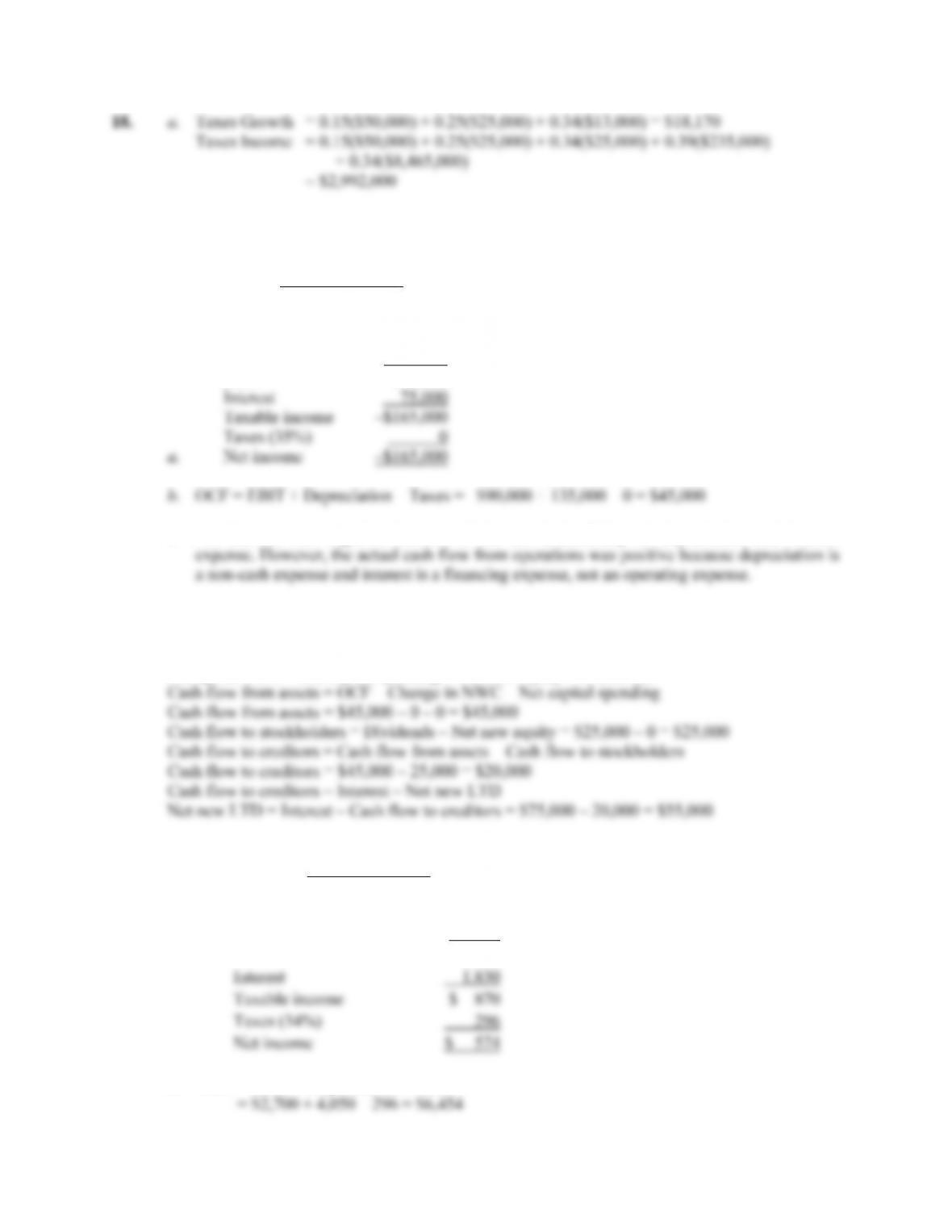B-10 SOLUTIONS
b. Each firm has a marginal tax rate of 34% on the next \$10,000 of taxable income, despite their
different average tax rates, so both firms will pay an additional \$3,400 in taxes.
19. Income Statement
Sales \$730,000
COGS 580,000
A&S expenses 105,000
Depreciation 135,000
EBIT –\$90,000
c. Net income was negative because of the tax deductibility of depreciation and interest
20. A firm can still pay out dividends if net income is negative; it just has to be sure there is sufficient
cash flow to make the dividend payments.
Change in NWC = Net capital spending = Net new equity = 0. (Given)
21. a.
Income Statement
Sales \$22,800
Cost of goods sold 16,050
Depreciation 4,050
EBIT \$ 2,700
b. OCF = EBIT + Depreciation – Taxes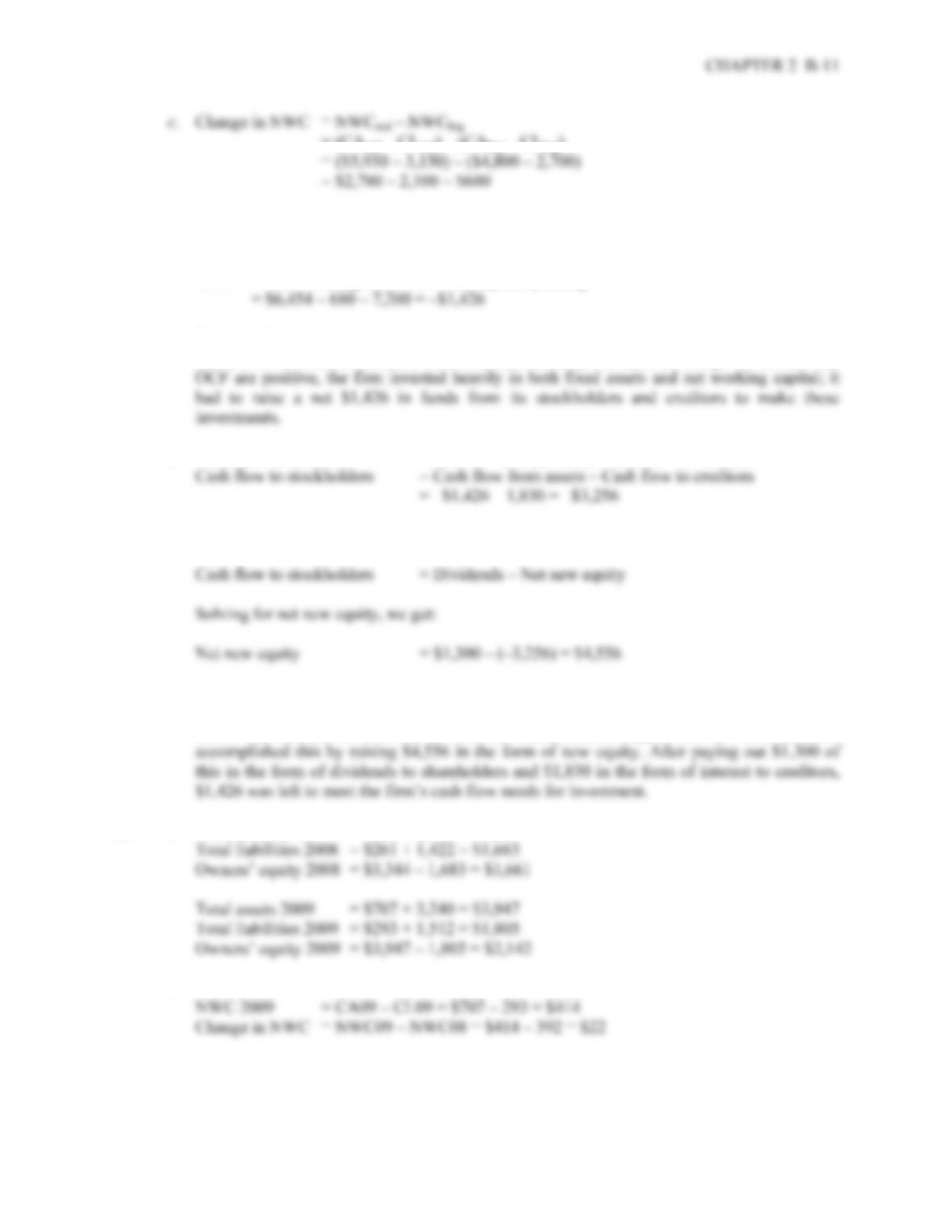= (CA
end – CLend) – (CAbeg – CLbeg)
Net capital spending = NFAend – NFAbeg + Depreciation
= \$16,800 – 13,650 + 4,050 = \$7,200
CFA = OCF – Change in NWC – Net capital spending
The cash flow from assets can be positive or negative, since it represents whether the firm
raised funds or distributed funds on a net basis. In this problem, even though net income and
d. Cash flow to creditors = Interest – Net new LTD = \$1,830 – 0 = \$1,830
We can also calculate the cash flow to stockholders as:
The firm had positive earnings in an accounting sense (NI > 0) and had positive cash flow
from operations. The firm invested \$680 in new net working capital and \$7,200 in new fixed
assets. The firm had to raise \$1,426 from its stakeholders to support this new investment. It
22. a. Total assets 2008 = \$653 + 2,691 = \$3,344
b. NWC 2008 = CA08 – CL08 = \$653 – 261 = \$392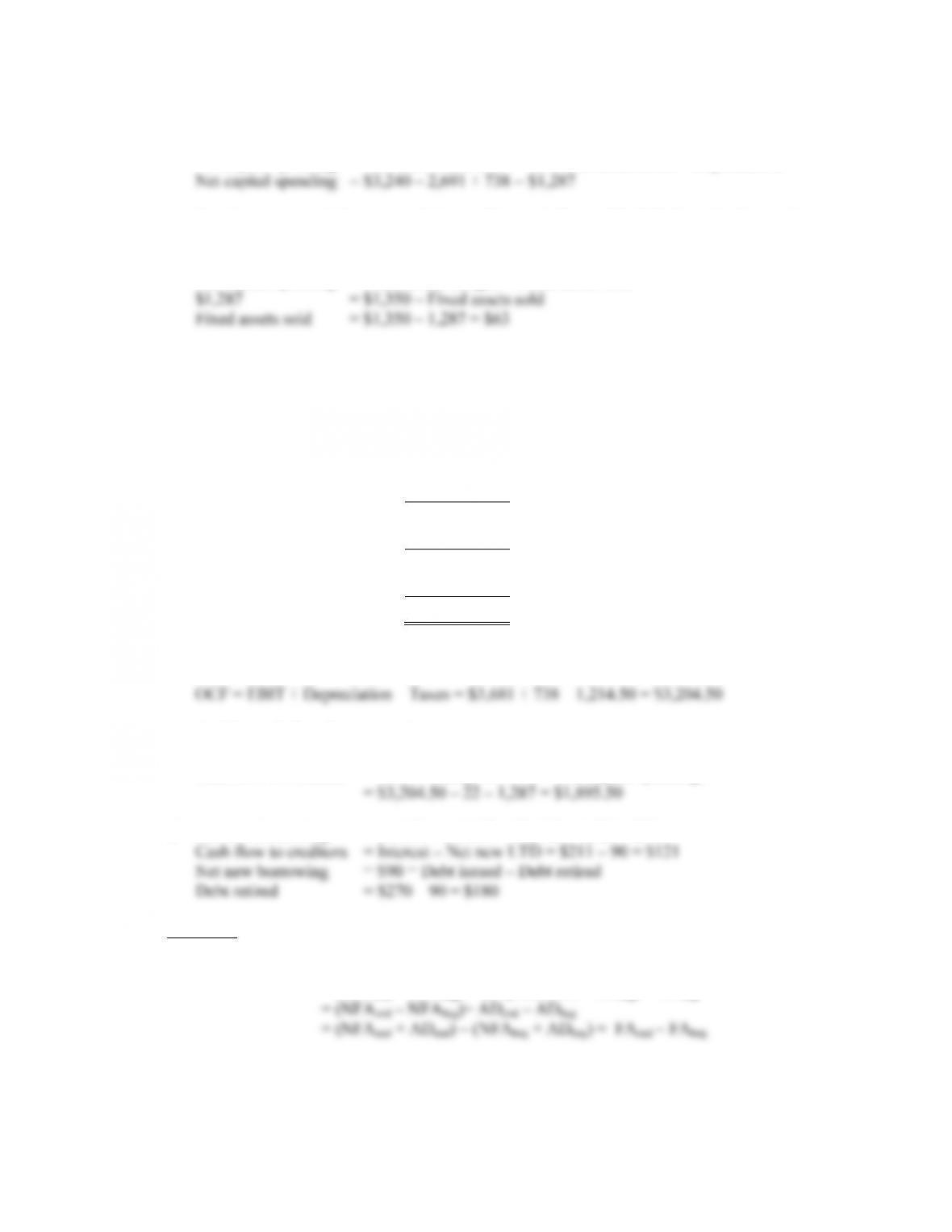B-12 SOLUTIONS
c. We can calculate net capital spending as:
Net capital spending = Net fixed assets 2009 – Net fixed assets 2008 + Depreciation
So, the company had a net capital spending cash flow of \$1,287. We also know that net
capital spending is:
Net capital spending = Fixed assets bought – Fixed assets sold
To calculate the cash flow from assets, we must first calculate the operating cash flow. The
income statement is:
Income Statement
Sales \$ 8,280.00
Costs 3,861.00
Depreciation expense 738 .00
EBIT \$3,681.00
Interest expense 211 .00
EBT \$3,470.00
Taxes (35%) 1,215.50
Net income \$2,256.50
So, the operating cash flow is:
And the cash flow from assets is:
Cash flow from assets = OCF – Change in NWC – Net capital spending.
d. Net new borrowing = LTD09 – LTD08 = \$1,512 – 1,422 = \$90
Challenge
23. Net capital spending = NFAend – NFAbeg + Depreciation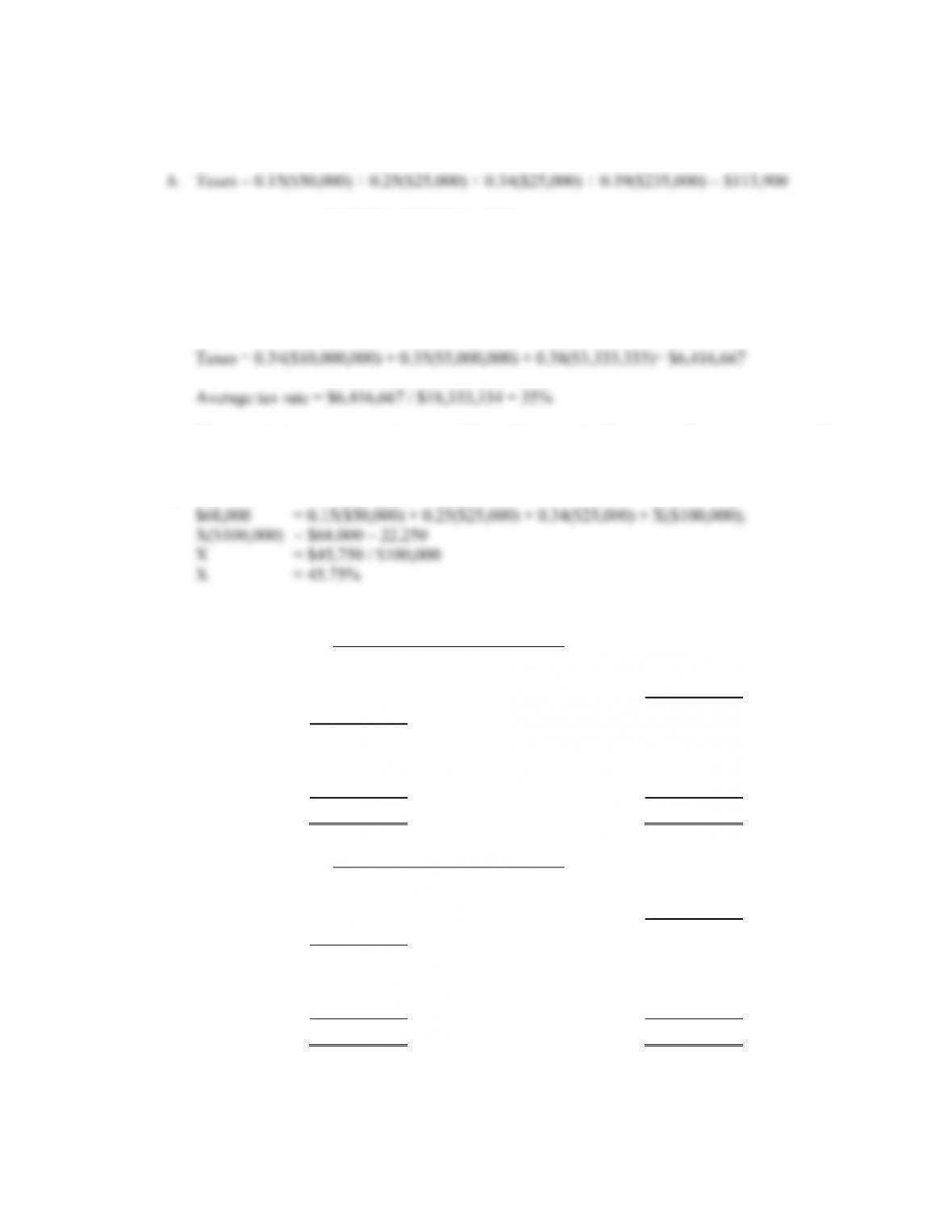CHAPTER 2 B-13
24. a. The tax bubble causes average tax rates to catch up to marginal tax rates, thus eliminating the
tax advantage of low marginal rates for high income corporations.
Average tax rate = \$113,900 / \$335,000 = 34%
The marginal tax rate on the next dollar of income is 34 percent.
For corporate taxable income levels of \$335,000 to \$10 million, average tax rates are equal to
marginal tax rates.
The marginal tax rate on the next dollar of income is 35 percent. For corporate taxable
income levels over \$18,333,334, average tax rates are again equal to marginal tax rates.
c. Taxes = 0.34(\$200,000) = \$68,000
25.
Balance sheet as of Dec. 31, 2008
Cash \$3,792 Accounts payable \$3,984
Accounts receivable 5,021 Notes payable 732
Inventory 8,927 Current liabilities \$4,716
Current assets \$17,740
Long-term debt \$12,700
Net fixed assets \$31,805 Owners' equity 32,129
Total assets \$49,545 Total liab. & equity \$49,545
Balance sheet as of Dec. 31, 2009
Cash \$4,041 Accounts payable \$4,025
Accounts receivable 5,892 Notes payable 717
Inventory 9,555 Current liabilities \$4,742
Current assets \$19,488
Long-term debt \$15,435
Net fixed assets \$33,921 Owners' equity 33,232
Total assets \$53,409 Total liab. & equity \$53,409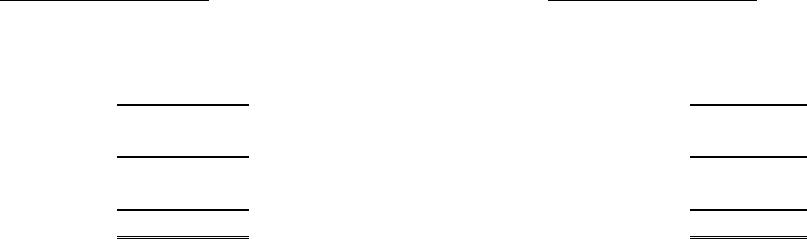B-14 SOLUTIONS
2008 Income Statement 2009 Income Statement
Sales \$7,233.00 Sales \$8,085.00
COGS 2,487.00 COGS 2,942.00
Other expenses 591.00 Other expenses 515.00
Depreciation 1,038.00 Depreciation 1,085.00
EBIT \$3,117.00 EBIT \$3,543.00
Interest 485.00 Interest 579.00
EBT \$2,632.00 EBT \$2,964.00
Taxes (34%) 894.88 Taxes (34%) 1,007.76
N
et income \$1,737.12 Net income \$1,956.24
Dividends \$882.00 Dividends \$1,011.00
26. OCF = EBIT + Depreciation – Taxes = \$3,543 + 1,085 – 1,007.76 = \$3,620.24
Change in NWC = NWCend – NWCbeg = (CA – CL) end – (CA – CL) beg
= (\$19,488 – 4,742) – (\$17,740 – 4,716)
= \$1,722
Net capital spending = NFAend – NFAbeg + Depreciation
= \$33,921 – 31,805 + 1,085 = \$3,201
Cash flow from assets = OCF – Change in NWC – Net capital spending
= \$3,620.24 – 1,722 – 3,201 = –\$1,302.76
Cash flow to creditors = Interest – Net new LTD
Net new LTD = LTDend – LTDbeg
Cash flow to creditors = \$579 – (\$15,435 – 12,700) = –\$2,156
Net new equity = Common stockend – Common stockbeg
Common stock + Retained earnings = Total owners’ equity
Net new equity = (OE – RE) end – (OE – RE) beg
= OEend – OEbeg + REbeg – REend
REend = REbeg + Additions to RE08
Net new equity = OEend – OEbeg + REbeg – (REbeg + Additions to RE08)
= OEend – OEbeg – Additions to RE
Net new equity = \$33,232 – 32,129 – 945.24 = \$157.76
CFS = Dividends – Net new equity
CFS = \$1,011 – 157.76 = \$853.24
As a check, cash flow from assets is –\$1,302.76.
CFA = Cash flow from creditors + Cash flow to stockholders
CFA = –\$2,156 + 853.24 = –\$1,302.76

## Trusted by Thousands ofStudents

Here are what students say about us.